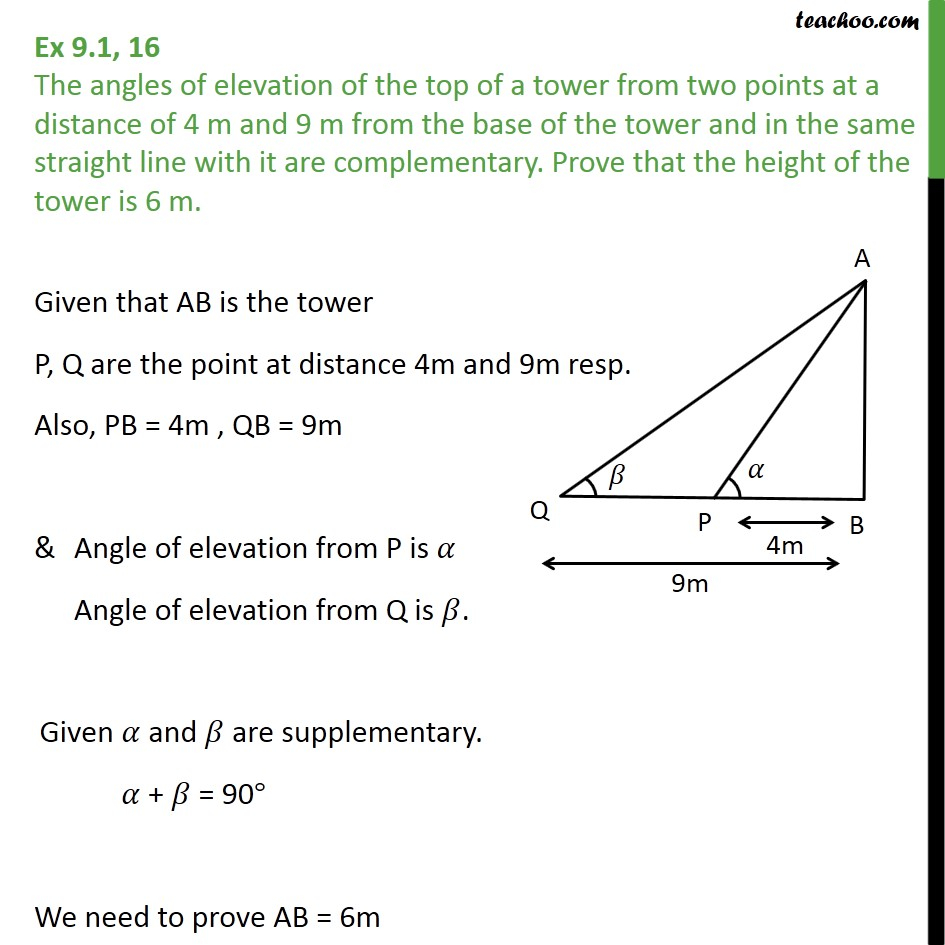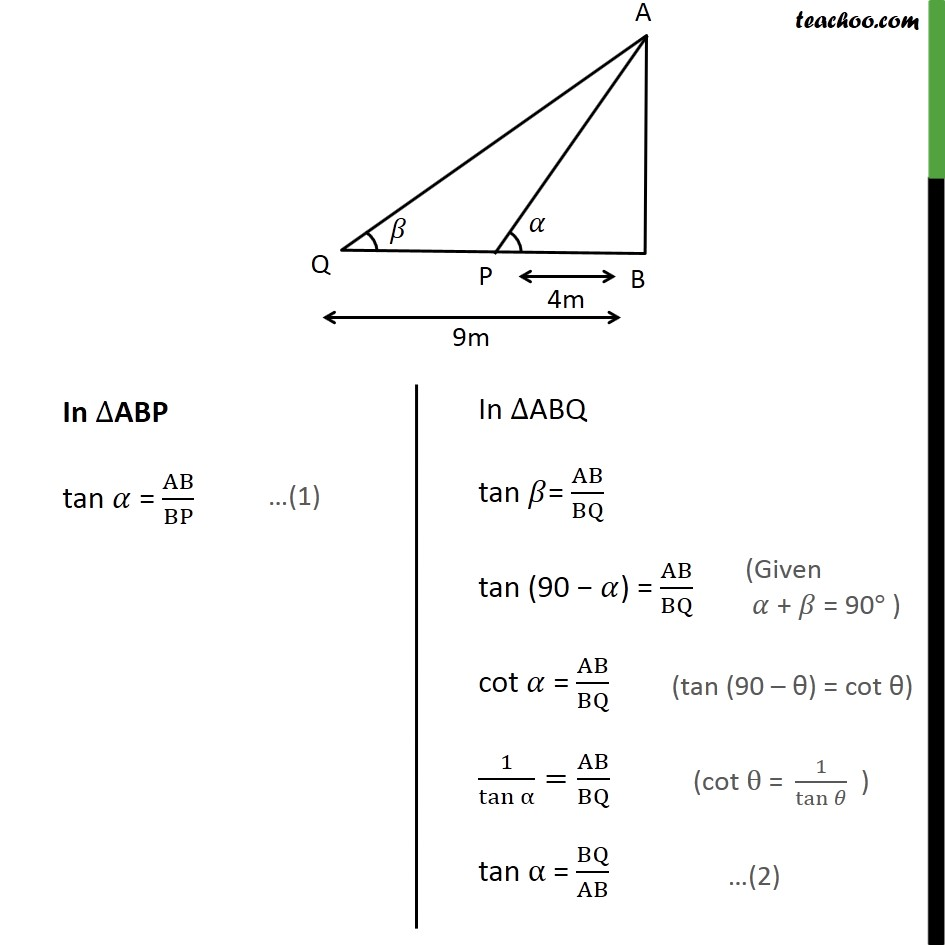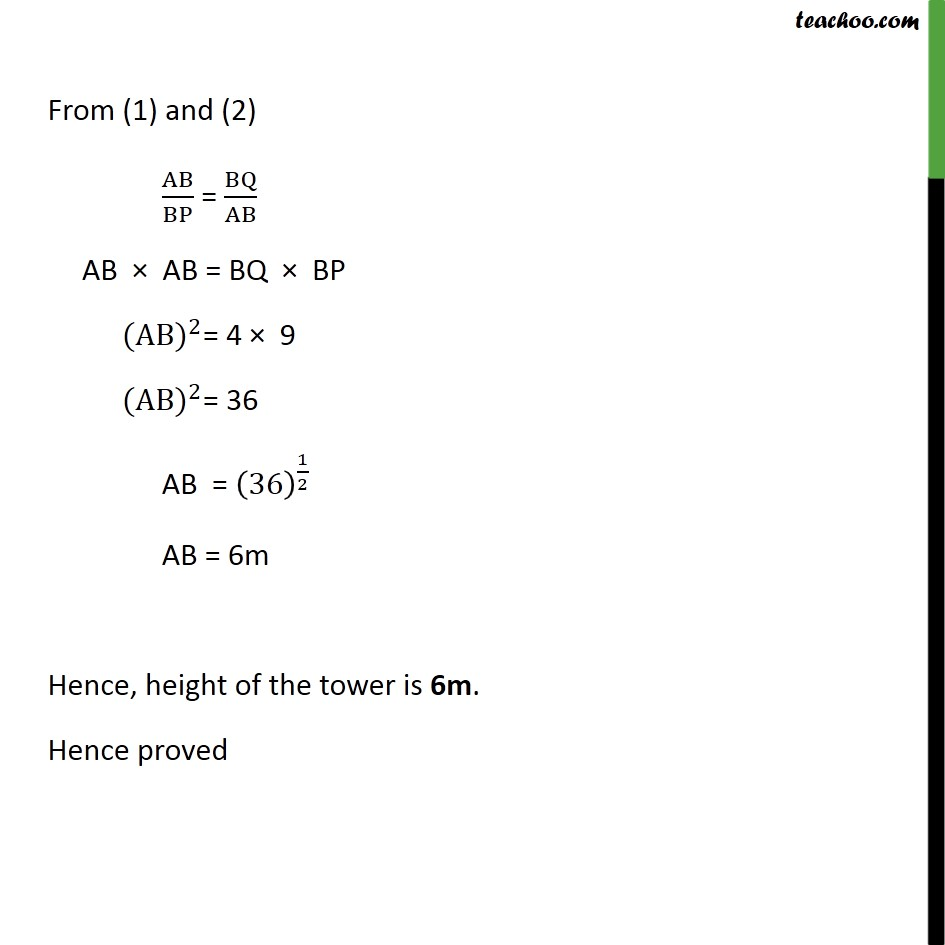1. Chapter 9 Class 10 Some Applications of Trigonometry (Term 2)
2. Concept wise
3. Questions easy to difficult

Transcript

Ex 9.1, 16 The angles of elevation of the top of a tower from two points at a distance of 4 m and 9 m from the base of the tower and in the same straight line with it are complementary. Prove that the height of the tower is 6 m. Given that AB is the tower P, Q are the point at distance 4m and 9m resp. Also, PB = 4m , QB = 9m & Angle of elevation from P is 𝛼 Angle of elevation from Q is 𝛽. Given 𝛼 and 𝛽 are supplementary. 𝛼 + 𝛽 = 90° We need to prove AB = 6m From (1) and (2) AB﷮BP﷯ = BQ﷮AB﷯ AB × AB = BQ × BP (AB)﷮2﷯= 4 × 9 (AB)﷮2﷯= 36 AB = 36﷯﷮ 1﷮2﷯﷯ AB = 6m Hence, height of the tower is 6m. Hence proved